Home » Polyhedra

# Volume of Polyhedra

Polyhedra (poly = many and hedra = faces), are a category of geometric shapes. Formed by flat faces, which are polygons with straight sides. Therefore, geometric shapes with curved surfaces are not considered polyhedra.

Something interesting about polyhedra is that for the majority of these shapes, Euler's formula can be applied. With this formula you can get the faces, vertices and edges.

Euler Formula: Faces + Vertices - Edges = 2

Then applying basic algebra you can get:

Faces = 2 - Vertices + Edges

Vertices = 2 - Faces + Edges

Edges = Vertices + Faces - 2

Down below you can select three-dimensional geometric shapes and inside you will find a description, the formula of the 3D geometric shape to calculate the volume and you can also use a volume calculator so you can calculate the volume of the automatically.

## Geometric shapes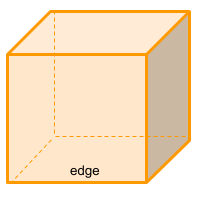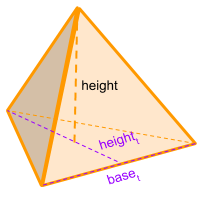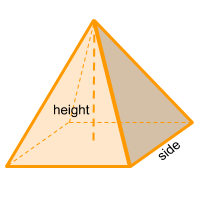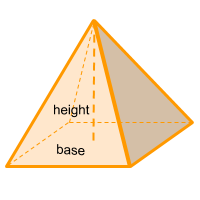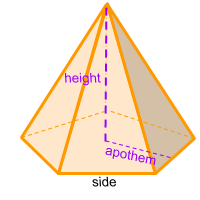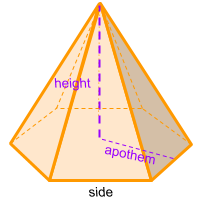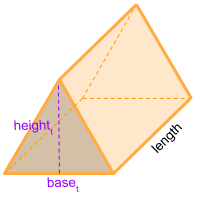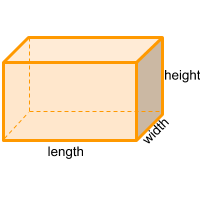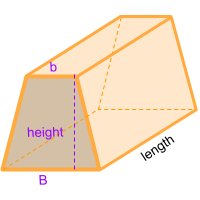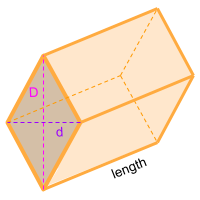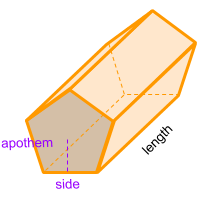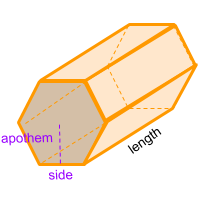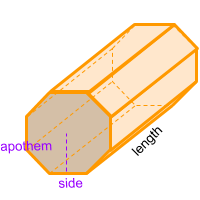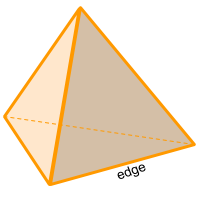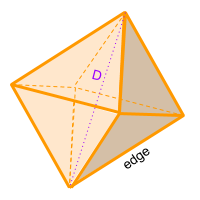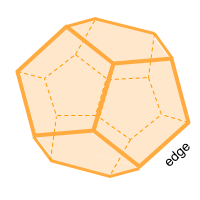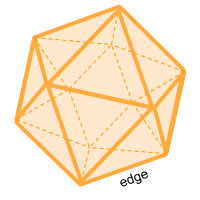Share